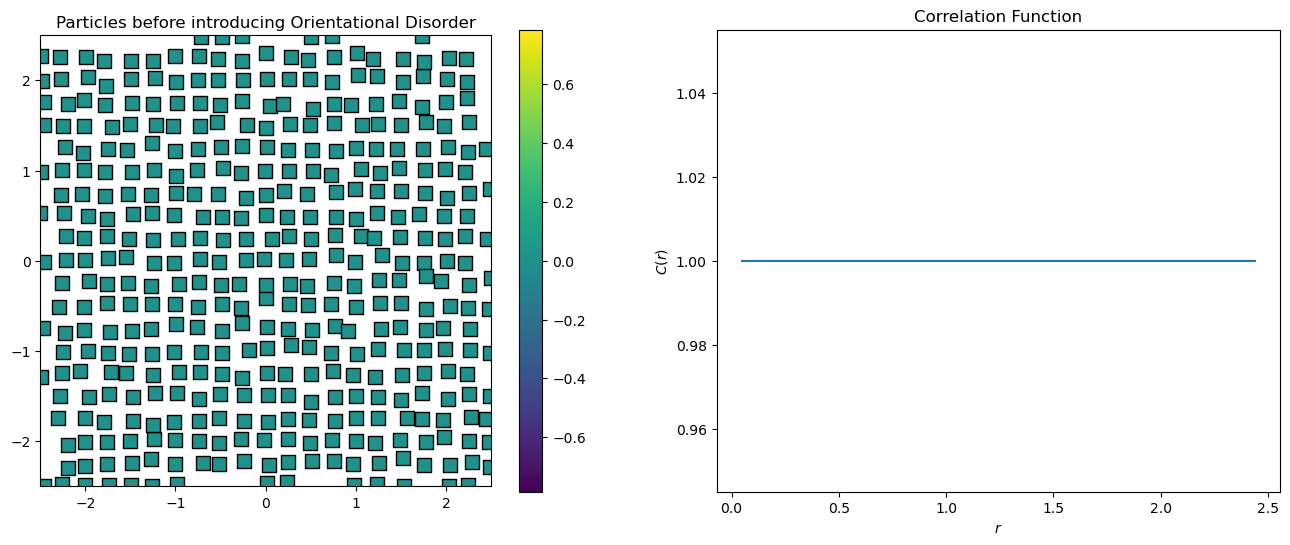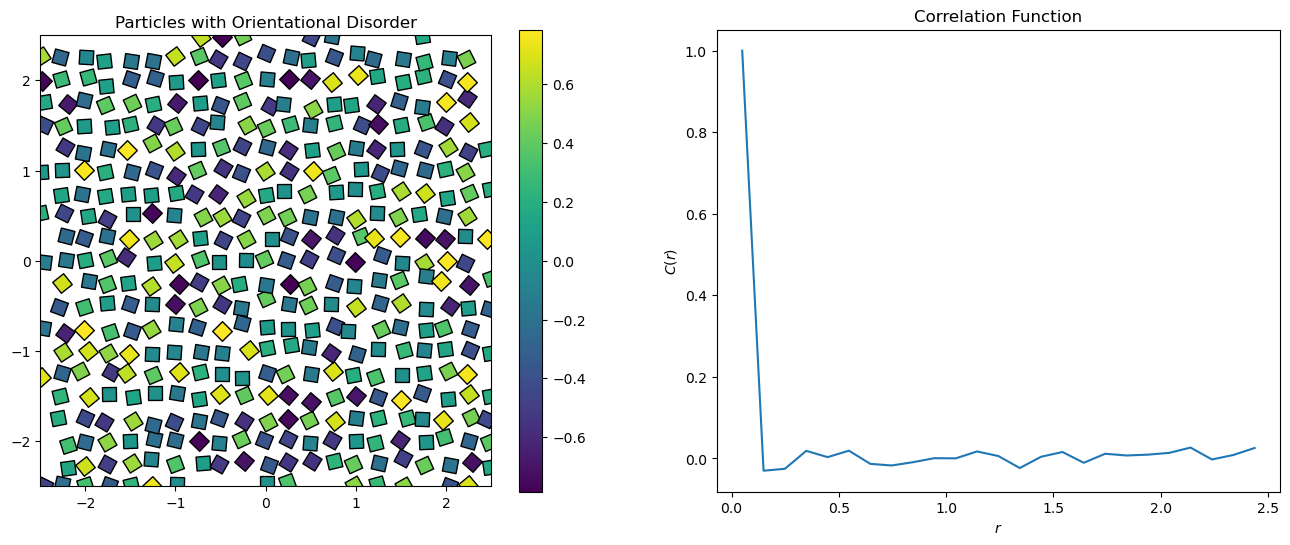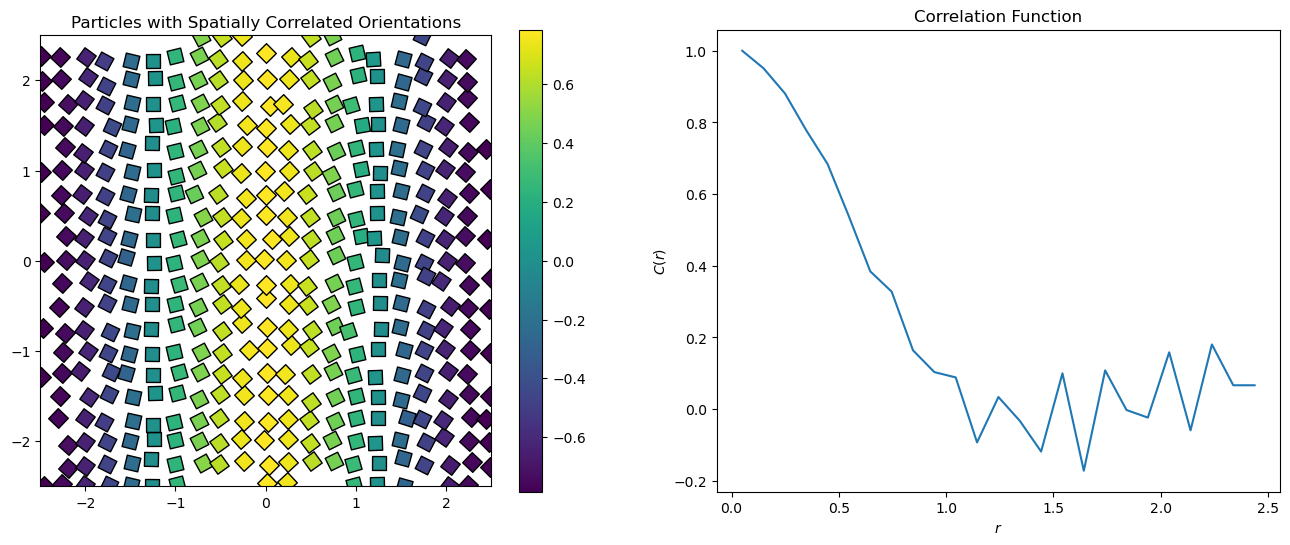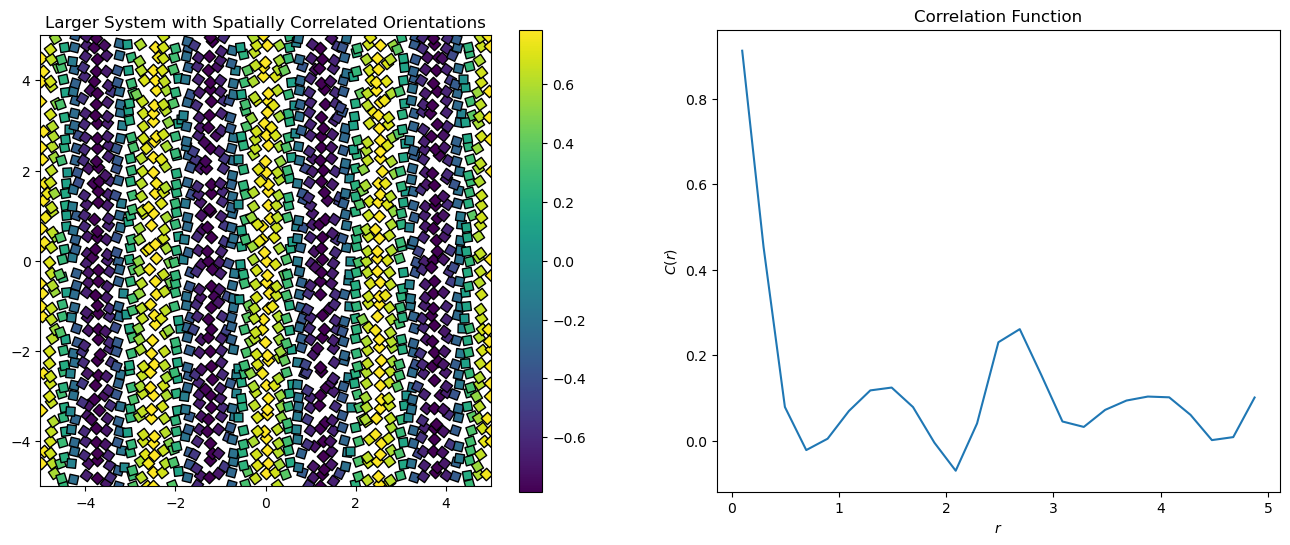# freud.density.CorrelationFunction#

## Orientational Ordering in 2D#

The freud.density module is intended to compute a variety of quantities that relate spatial distributions of particles with other particles. This example shows how correlation functions can be used to measure orientational order in 2D.

:

import freud
import matplotlib.cm
import matplotlib.pyplot as plt
import numpy as np
from matplotlib.colors import Normalize


This helper function will make plots of the data we generate in this example.

:

def plot_data(title, points, angles, values, box, cf, s=200):
cmap = matplotlib.cm.viridis
norm = Normalize(vmin=-np.pi / 4, vmax=np.pi / 4)
plt.figure(figsize=(16, 6))
plt.subplot(121)
for point, angle, value in zip(points, angles, values):
plt.scatter(
point,
point,
edgecolor="k",
c=[cmap(norm(angle))],
s=s,
)
plt.title(title)
plt.gca().set_xlim([-box.Lx / 2, box.Lx / 2])
plt.gca().set_ylim([-box.Ly / 2, box.Ly / 2])
plt.gca().set_aspect("equal")
sm = plt.cm.ScalarMappable(cmap="viridis", norm=norm)
sm.set_array(angles)
plt.colorbar(sm)
plt.subplot(122)
plt.title("Orientation Spatial Autocorrelation Function")
cf.plot(ax=plt.gca())
plt.xlabel(r"$r$")
plt.ylabel(r"$C(r)$")
plt.show()


First, let’s generate a 2D structure with perfect orientational order and slight positional disorder (the particles are not perfectly on a grid, but they are perfectly aligned). The color of the particles corresponds to their angle of rotation, so all the particles will be the same color to begin with.

We create a freud.density.CorrelationFunction object to compute the correlation functions. Given a particle orientation $$\theta$$, we compute its complex orientation value (the quantity we are correlating) as $$s = e^{4i\theta}$$, to account for the fourfold symmetry of the particles. We will compute the correlation function $$C(r) = \left\langle s^*_1(0) \cdot s_2(r) \right\rangle$$ by taking the average over all particle pairs and binning the results into a histogram by the distance $$r$$ between the particles.

When we compute the correlations between particles, the complex conjugate of the values array is used internally for the query points. This way, if $$\theta_1$$ is close to $$\theta_2$$, then we get $$\left(e^{4i\theta_1}\right)^* \cdot \left(e^{4i\theta_2}\right) = e^{4i(\theta_2-\theta_1)} \approx e^0 = 1$$.

This system has perfect spatial correlation of the particle orientations, so we see $$C(r) = 1$$ for all values of $$r$$.

:

def make_particles(L, repeats):
uc = freud.data.UnitCell.square()
return uc.generate_system(
num_replicas=repeats, scale=L / repeats, sigma_noise=5e-3 * L
)

# Make a small system
box, points = make_particles(L=5, repeats=20)

# All the particles begin with their orientation at 0
angles = np.zeros(len(points))
values = np.array(np.exp(angles * 4j))

# Create the CorrelationFunction compute object and compute the correlation function
cf = freud.density.CorrelationFunction(bins=25, r_max=box.Lx / 2.01)
cf.compute(
system=(box, points), values=values, query_points=points, query_values=values
)

plot_data(
"Particles before introducing Orientational Disorder",
points,
angles,
values,
box,
cf,
)Now we will generate random angles from $$-\frac{\pi}{4}$$ to $$\frac{\pi}{4}$$, which orients our squares randomly. The four-fold symmetry of the squares means that the space of unique angles is restricted to a range of $$\frac{\pi}{2}$$. Again, we compute a complex value for each particle, $$s = e^{4i\theta}$$.

Because we have purely random orientations, we expect no spatial correlations in the plot above. As we see, $$C(r) \approx 0$$ for all $$r$$.

:

# Change the angles to values randomly drawn from a uniform distribution
angles = np.random.uniform(-np.pi / 4, np.pi / 4, size=len(points))
values = np.exp(angles * 4j)

# Recompute the correlation functions
cf.compute(
system=(box, points), values=values, query_points=points, query_values=values
)

plot_data("Particles with Orientational Disorder", points, angles, values, box, cf)The plot below shows what happens when we intentionally introduce a correlation length by adding a spatial pattern to the particle orientations. At short distances, the correlation is very high. As $$r$$ increases, the oppositely-aligned part of the pattern some distance away causes the correlation to drop.

:

# Use angles that vary spatially in a pattern
angles = np.pi / 4 * np.cos(2 * np.pi * points[:, 0] / box.Lx)
values = np.exp(angles * 4j)

# Recompute the correlation functions
cf.compute(
system=(box, points), values=values, query_points=points, query_values=values
)

plot_data(
"Particles with Spatially Correlated Orientations", points, angles, values, box, cf
)In the larger system shown below, we see the spatial autocorrelation rise and fall with damping oscillations.

:

# Make a large system
box, points = make_particles(L=10, repeats=40)

# Use angles that vary spatially in a pattern
angles = np.pi / 4 * np.cos(8 * np.pi * points[:, 0] / box.Lx)
values = np.exp(angles * 4j)

# Create a CorrelationFunction compute object
cf = freud.density.CorrelationFunction(bins=25, r_max=box.Lx / 2.01)
cf.compute(
system=(box, points), values=values, query_points=points, query_values=values
)

plot_data(
"Larger System with Spatially Correlated Orientations",
points,
angles,
values,
box,
cf,
s=80,
)